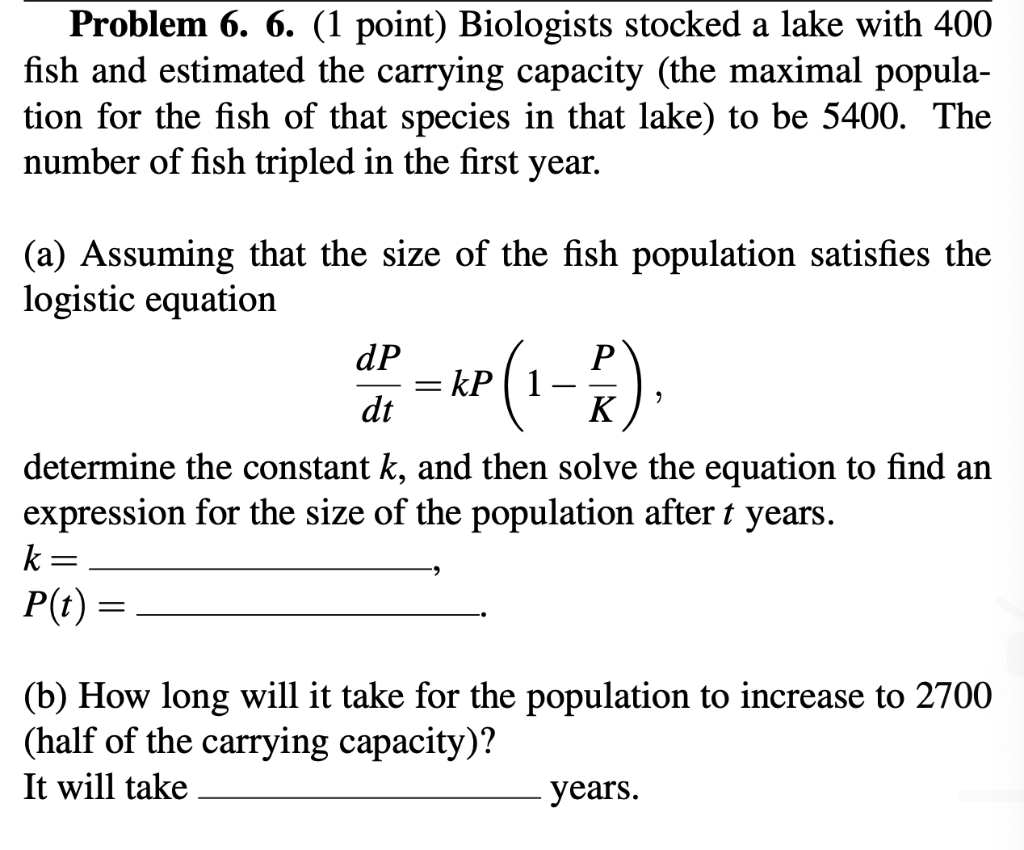# (Solved): Problem 6. 6. (1 point) Biologists stocked a lake with 400 fish and estimated the carrying capacit ...Problem 6. 6. (1 point) Biologists stocked a lake with 400 fish and estimated the carrying capacity (the maximal population for the fish of that species in that lake) to be 5400. The number of fish tripled in the first year. (a) Assuming that the size of the fish population satisfies the logistic equation $\frac{d P}{d t}=k P\left(1-\frac{P}{K}\right),$ determine the constant $$k$$, and then solve the equation to find an expression for the size of the population after $$t$$ years. $$k=$$ $$P(t)=$$ (b) How long will it take for the population to increase to 2700 (half of the carrying capacity)? It will take years.

We have an Answer from Expert